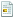July 06, 2022, 06:49:47 am

## LPC 1343 GPIO INTERRUPT

Started by e3402, June 23, 2015, 02:20:23 pm

#### e3402

#####June 23, 2015, 02:20:23 pmLast Edit: June 23, 2015, 02:36:24 pm by e3402
Hi,
I could not find how to use  gpio interrupt for lpc 1343 and I am using iar.I have added interrupt function (is it true?) and configured pins that I want to use.My aim is to turn on first led, then after pressing user's button to turn on second led when the first one is turned off.I am a beginner one, so sending whole code please make corrections on it.In addition to that issue, I could not find helpful example code for lpc 1343 what is your suggestion to develop myself? Here is the code.Thanks.

#include <nxp/iolpc1343.h>

#define LED_0_ON                GPIO3DATA_bit.P3_0=0;
#define LED_0_OFF               GPIO3DATA_bit.P3_0=1;
#define LED_1_ON                GPIO3DATA_bit.P3_1=0;
#define LED_1_OFF               GPIO3DATA_bit.P3_1=1;
#define Button_1_pressed        !(GPIO2DATA_bit.P2_9)

void NVIC_IntEnable(unsigned long IntNumber);

int delay = 400000;
void main()
{

volatile unsigned long int i;

IOCON_PIO3_0_bit.FUNC = 0;
IOCON_PIO3_0_bit.MODE = 0;
IOCON_PIO3_0_bit.HYS = 0;

IOCON_PIO3_1_bit.FUNC = 0;
IOCON_PIO3_1_bit.MODE = 0;
IOCON_PIO3_1_bit.HYS = 0;

GPIO3DIR_bit.P3_0 = 1;
GPIO3DIR_bit.P3_1 = 1;

IOCON_PIO2_9=0;
GPIO2DIR_bit.P2_9 = 0;
IOCON_PIO2_9_bit.MODE=0x2;
GPIO2IE_bit.P2_9=1;
GPIO2IS_bit.P2_9=0;
GPIO2IEV_bit.P2_9 = 1;

for(i=0; i<256 ; i++)
NVIC_IntEnable(i);
while(1)
{

GPIO3DATA_bit.P3_0=0;
for(i=0;i<delay;i++);
GPIO3DATA_bit.P3_0=1;
for(i=0;i<delay;i++);

}

}

void NVIC_IntEnable(unsigned long IntNumber)
{
volatile unsigned long * pNVIC_SetEn = &SETENA0;

//assert((NVIC_WAKE_UP0 <= IntNumber) && (NVIC_PIO_0 >= IntNumber));
IntNumber -= NVIC_WAKE_UP2; // (IntNumber = NVIC_CT32B0 = 59) - (NVIC_WAKE_UP0 = 16) = 43(NVIC_WAKE_UP27)
pNVIC_SetEn += IntNumber/32;
*pNVIC_SetEn = (1UL<<(IntNumber%32));

}

void PIOINT2_IRQHandler(void)
{

// unsigned long int i;
delay /= 2;
/* while(1)
{

GPIO3DATA_bit.P3_1=0;
for(i=0;i<100000;i++);
GPIO3DATA_bit.P3_1=1;
for(i=0;i<100000;i++);

}*/
}